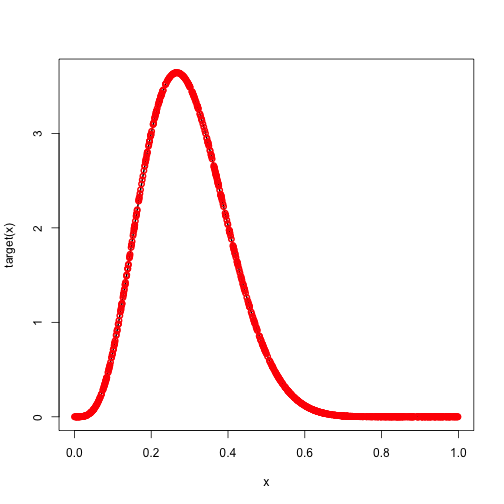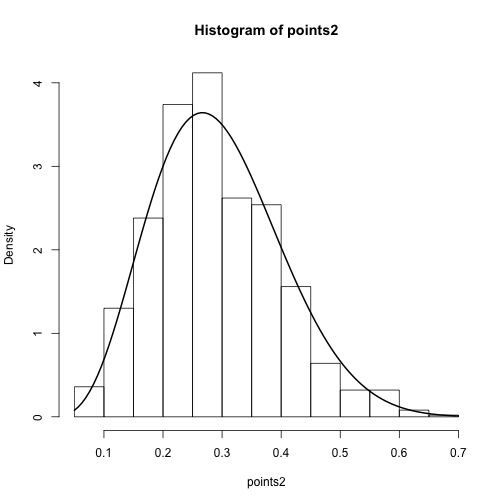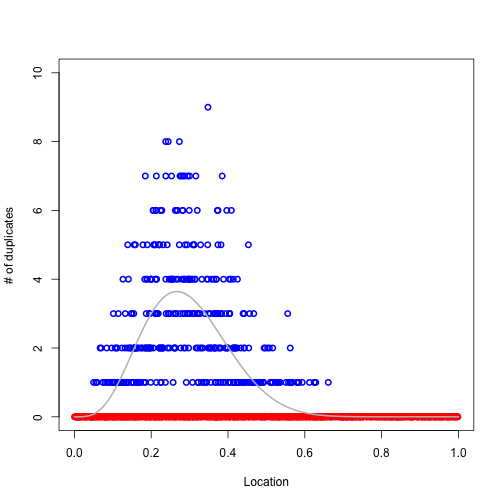Continuing with our rejection sampling example, we now turn to importance sampling. We still have a target distribution f(x) and a proposal distribution q(x). But instead of throwing away samples like we did in rejection sampling, we keep all the samples but give them weights. The weights are proportional to the ratio of the target to the proposal. They are only proportional because we renormalize them to sum to 1.

Set up the target and proposal densities and sample.

So far, everything is the same as rejection sampling. Now we calculate the weights.Since our proposal is the uniform distribution, the unnormalized weights exactly match the density of our target. We can use this weighted sample for any purpose, e.g. estimation of expectations or densities. But sometimes it is more convenient to have an unweighted sample. If so, we can resample from our weights with replacement to obtain an unweighted sample.

These unweighted samples should look like our target density.But notice that there are duplications in this sample.

The plot below shows that the duplicated points are more often found where the target density is high.